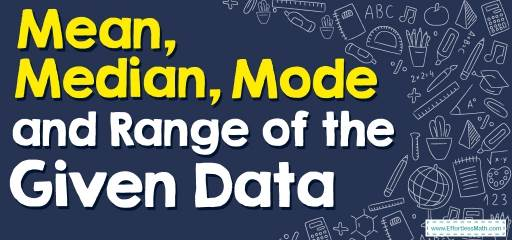# How to Find Mean, Median, Mode, and Range of the Given Data? (+FREE Worksheet!)

Here you can learn how to find and calculate the Mean, Median, Mode, and Range of the Given Data.## Step by step guide to Find Mean, Median, Mode, and Range of the Given Data

• Mean: $$\frac{sum \ of \ the \ data}{total \ number \ of \ data \ entires}$$
• Mode: value in the list that appears most often
• Median: the middle value of the numbers.
• Range: the difference of the largest value and smallest value in the list

The Absolute Best Book to Ace Algebra I

### Mean, Median, Mode, and Range of the Given Data – Example 1:

What is the mode of these numbers? $$18,12,8,5,3,2,0,2$$

Solution:

Mode: value in the list that appears most often.
Therefore: mode is $$2$$

### Mean, Median, Mode, and Range of the Given Data – Example 2:

What is the median of these numbers? $$2,7,11,6,13,16,3$$

Solution:

Write the numbers in order: $$2,3,6,7,11,13,16$$
The Median is the number in the middle. Therefore, the median is $$7$$.

### Mean, Median, Mode, and Range of the Given Data – Example 3:

What is the median of these numbers? $$4,9,13,8,15,18,5$$

Solution:

Write the numbers in order: $$4,5,8,9,13,15,18$$
The Median is the number in the middle. Therefore, the median is $$9$$.

Best Algebra Prep Resource

### Mean, Median, Mode, and Range of the Given Data – Example 4:

What is the range of these numbers? $$22,16,12,9,7,6,4,6$$

Solution:

The range is the difference between the largest value and the smallest value.
Therefore: range is $$22-4=18$$

## Exercises for Finding Mean, Median, Mode, and Range of the Given Data

### Find Mean, Median, Mode, and Range of the Given Data.

1. $$\color{blue}{7, 2, 5, 1, 1, 2}$$
2. $$\color{blue}{2, 2, 2, 3, 6, 3, 7, 4 }$$
3. $$\color{blue}{9, 4, 3, 1, 7, 9, 4, 6, 4 }$$
4. $$\color{blue}{8, 4, 2, 4, 3, 2, 4, 5}$$
5. $$\color{blue}{8, 5, 7, 5, 7, 9, 8}$$
6. $$\color{blue}{5, 1, 4, 4, 9, 2, 9, 2, 5, 1}$$

1. $$\color{blue}{mean: 3, \ median: 2, \ mode: 1, 2, \ range: 6}$$
2. $$\color{blue}{mean: 3.625, \ median: 3, \ mode: 2, \ range: 5}$$
3. $$\color{blue}{mean: 5.22, \ median: 4, \ mode: 4, \ range: 8}$$
4. $$\color{blue}{mean: 4, \ median: 4, \ mode: 4, \ range: 6}$$
5. $$\color{blue}{mean: 7, \ median: 7, \ mode: 5, 7, 8, \ range: 4}$$
6. $$\color{blue}{mean: 4.2, \ median: 4, \ mode: 1,2,4,5,9, \ range: 8}$$

The Best Books to Ace Algebra

### What people say about "How to Find Mean, Median, Mode, and Range of the Given Data? (+FREE Worksheet!) - Effortless Math: We Help Students Learn to LOVE Mathematics"?

No one replied yet.

X
30% OFF

Limited time only!

Save Over 30%

SAVE $5 It was$16.99 now it is \$11.99